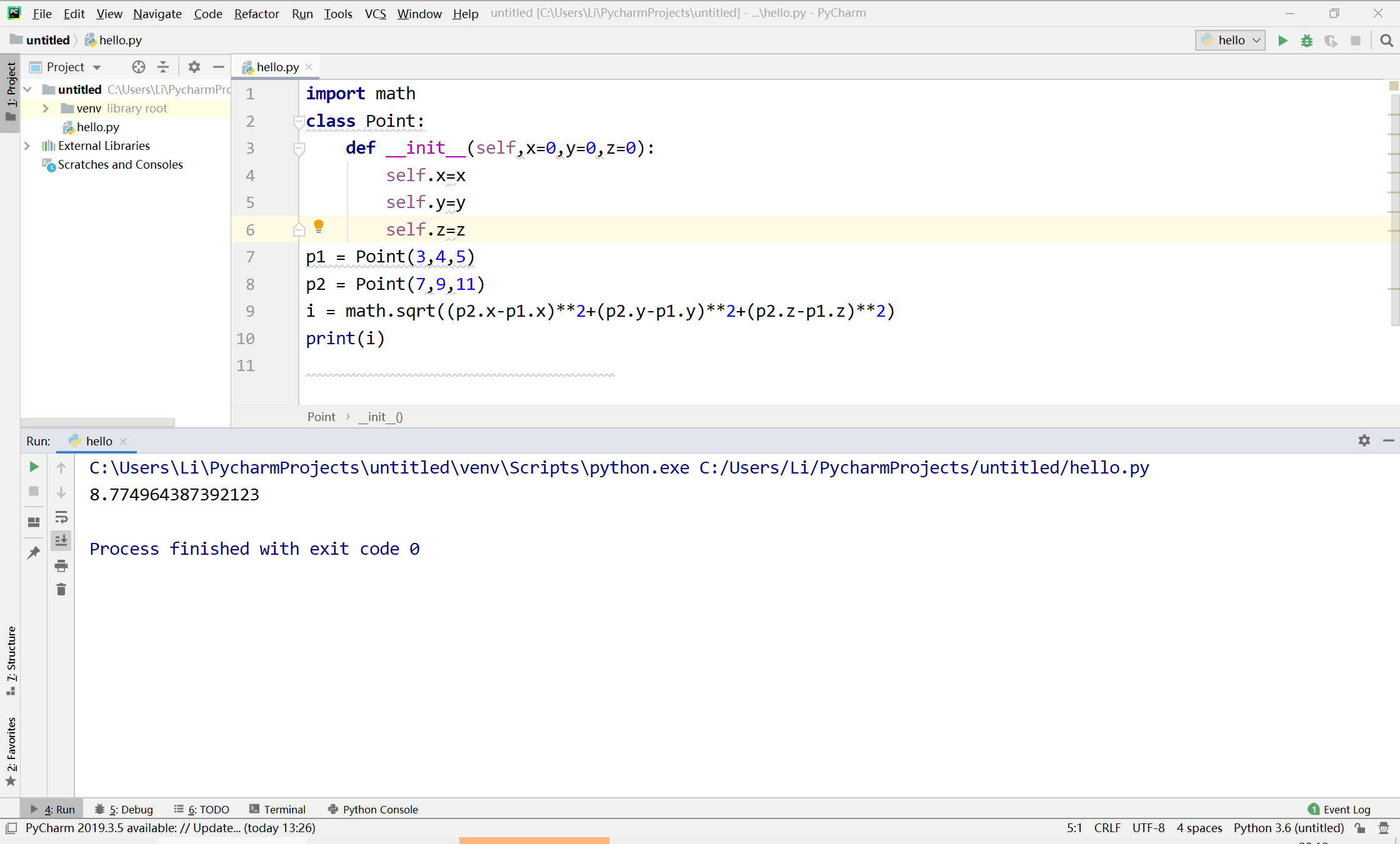• 如下所示：from mpl_toolkits.mplot3d import axes3dimport matplotlib.pyplot as plt# 打开画图窗口1，在三维空间中绘图fig = plt.figure(1)ax = fig.gca(projection='3d')# 给出点(0，0，0)和(100，200，300)x = ...

如下所示：

from mpl_toolkits.mplot3d import axes3d

import matplotlib.pyplot as plt

# 打开画图窗口1，在三维空间中绘图

fig = plt.figure(1)

ax = fig.gca(projection='3d')

# 给出点(0，0，0)和(100，200，300)

x = [0, 100]

y = [0, 200]

z = [0, 300]

# 将数组中的前两个点进行连线

figure = ax.plot(x, y, z, c='r')

plt.show()

运行结果如下在二维空间中，我们只需要修改

fig.gca(projection='3d')为fig.gca()

示例如下：

from mpl_toolkits.mplot3d import axes3d

import matplotlib.pyplot as plt

# 打开画图窗口1，在三维空间中绘图

fig = plt.figure(1)

ax = fig.gca()

# 给出点(0，0，0)和(100，200，300)

x = [0, 100]

y = [0, 200]

z = [0, 300]

# 将数组中的前两个点进行连线

figure = ax.plot(x, y ,c='r')

plt.show()

结果如下：以上这篇python 画二维、三维点之间的线段实现方法就是小编分享给大家的全部内容了，希望能给大家一个参考，也希望大家多多支持我们。

本文标题: python 画二维、三维点之间的线段实现方法

本文地址: http://www.cppcns.com/jiaoben/python/264793.html

展开全文• class Point: #定义一个 def __init__(self,x=0,y=0,z=0): #初始化x，y，z self.x=x self.y=y self.z=z def getx(self): #得到x坐标 return self.x def gety(self): #得到y坐标 return self.y def g

代码：

import math  #导入math
class Point:  #定义一个类
def __init__(self,x=0,y=0,z=0):  #初始化x，y，z
self.x=x
self.y=y
self.z=z
def getx(self):  #得到x坐标
return self.x
def gety(self):  #得到y坐标
return self.y
def getz(self):  #得到z坐标
return self.z
class Getlen:  #定义一个类
def __init__(self,p1,p2):
self.x=p1.getx() -  p2.getx()
self.y=p1.gety() -  p2.gety()
self.z=p1.getz() -  p2.getz()
self.len=math.sqrt(self.x**2+self.y**2+self.z**2)  #计算公式
def getlen(self):
return self.len  #得到距离·1
p1 = Point(3,4,5)  #设置p1坐标
p2 = Point(7,9,11)  #设置p2坐标
l = Getlen(p1,p2)  #定义对象
print(l.getlen())  #输出

import math
class Point:
def __init__(self,x=0,y=0,z=0):
self.x=x
self.y=y
self.z=z
p1 = Point(3,4,5)
p2 = Point(7,9,11)
print(math.sqrt((p2.x-p1.x)**2+(p2.y-p1.y)**2+(p2.z-p1.z)**2))
print（i）

运行结果：展开全文python
• //4、有Distance类Point类，将Distance类定义Point类的友元实现计算两点之间距离。 #include"iostream" #include"math.h" using namespace std; class point { private: float x,y; public: point(int a,...

//4、有Distance类和Point类，将Distance类定义为Point类的友元类来实现计算两点之间距离。
#include"iostream"
#include"math.h"
using namespace std;
class point
{
private:
float x,y;
public:
point(int a,int b):x(a),y(b){}
friend class distance1;
};
class distance1
{

public:
void print(point p1,point p2);

};
void distance1::print(point p1,point p2)
{

cout<<"两点之间的距离："<<endl;
cout<<(sqrt((p1.x-p2.x)*(p1.x-p2.x)+(p1.y-p2.y)*(p1.y-p2.y)));
}
int main()
{
point pp1(2,3),pp2(4,5);
distance1 d1;
d1.print(pp1,pp2);
return 0;

}

展开全文c++
• Python两点之间的直线距离(2种实现方法)方法一：#导入math包import math#定义点的函数class Point:def __init__(self,x=0,y=0):self.x=xself.y=ydef getx(self):return self.xdef gety(self):return self.y#定义...

Python求两点之间的直线距离(2种实现方法)

方法一：

#导入math包

import math

#定义点的函数

class Point:

def __init__(self,x=0,y=0):

self.x=x

self.y=y

def getx(self):

return self.x

def gety(self):

return self.y

#定义直线函数

class Getlen:

def __init__(self,p1,p2):

self.x=p1.getx()-p2.getx()

self.y=p1.gety()-p2.gety()

#用math.sqrt（）求平方根

self.len= math.sqrt((self.x**2)+(self.y**2))

#定义得到直线长度的函数

def getlen(self):

return self.len

#设置点p1的坐标为（0,0）

p1=Point(0,0)

#设置点p2的坐标为（3,4）

p2=Point(3,4)

#定义对象

l=Getlen(p1,p2)

#获取两点之间直线的长度

d=l.getlen())

方法二：

import numpy as np

import math

p1=np.array([0,0])

p2=np.array([1000,2000])

p3=p2-p1

p4=math.hypot(p3,p3)

print(p4)

以上这篇Python求两点之间的直线距离(2种实现方法)就是小编分享给大家的全部内容了，希望能给大家一个参考，也希望大家多多支持我们。

时间： 2019-07-05

详细: 1.闵可夫斯基距离(Minkowski Distance) 2.欧氏距离(Euclidean Distance) 3.曼哈顿距离(Manhattan Distance) 4.切比雪夫距离(Chebyshev Distance) 5.夹角余弦(Cosine) 6.汉明距离(Hamming distance) 7.杰卡德相似系数(Jaccard similarity coefficient) 8.贝叶斯公式 1.闵氏距离的定义: 两个n维变量A(x11,x12,-,x1n)与 B(x21,x前言 之前一直写不出来,这周周日花了一下午终于弄懂了, 顺便放博客里,方便以后忘记了再看看. 要实现的是输入一张 图,起点,终点,输出起点和终点之间的最短路径. 广度优先搜索 适用范围: 无权重的图,与深度优先搜索相比,深度优先搜索法占内存少但速度较慢,广度优先搜索算法占内存多但速度较快 复杂度: 时间复杂度为O(V+E),V为顶点数,E为边数 思路 广度优先搜索是以层为顺序,将某一层上的所有节点都搜索到了之后才向下一层搜索: 比如下图: 从0结点开始搜索的话,一开始是0.将0加入队列中: 然后

复制代码 代码如下: /** * 计算两点之间距离 * @param _lat1 - start纬度 * @param _lon1 - start经度 * @param _lat2 - end纬度 * @param _lon2 - end经度 * @return km(四舍五入) */public static double getDistance(double _lat1,double _lon1, double _lat2,double _lon2){ double lat1 = (Math

SQL SERVER 根据地图经纬度计算距离的公式 复制代码 代码如下: go --创建经纬度距离计算函数 CREATEFUNCTION [dbo].[fnGetDistance] --LatBegin 开始经度 --LngBegin 开始维度 (@LatBegin REAL, @LngBegin REAL, @LatEnd REAL, @LngEnd REAL) RETURNSFLOAT AS BEGIN --距离(千米) DECLARE @Distance REAL DECLARE @EAR

这是一个不错的示例,直接贴代码,首先要知道纬度值.经度值 /** * @desc 根据两点间的经纬度计算距离 * @param float $lat 纬度值 * @param float$lng 经度值 */ function getDistance($lat1,$lng1, $lat2,$lng2) { earthRadius = 6367000; //approximate radius of earth in meters /* Convert these degrees to r 经度指示南北方向,纵向纬度指示东西方向,横向 获取经纬度 使用GPS权限: 复制代码 代码如下: Android提供LocationManager和Location,可以方便的获得经纬度.海拔等位置.使用LocationManager来获得位置管理类,从而可以获得历史GPS信息以及位 本文实例讲述了Python基于高斯消元法计算线性方程组.分享给大家供大家参考,具体如下: #!/usr/bin/env python # coding=utf-8 # 以上的信息随自己的需要改动吧 def print_matrix( info, m ): # 输出矩阵 i = 0; j = 0; l = len(m) print info for i in range( 0, len( m ) ): for j in range( 0, len( m[i] ) ): if( j == l ): 根据两点经纬度计算距离 这些经纬线是怎样定出来的呢?地球是在不停地绕地轴旋转(地轴是一根通过地球南北两极和地球中心的假想线),在地球中腰画一个与地轴垂直的大圆圈,使圈上的每一点都和南北两极的距离相等,这个圆圈就叫作"赤道".在赤道的南北两边,画出许多和赤道平行的圆圈,就是"纬圈":构成这些圆圈的线段,叫做纬线.我们把赤道定为纬度零度,向南向北各为90度,在赤道以南的叫南纬,在赤道以北的叫北纬. 北极就是北纬90度,南极就是南纬90度.纬度的高低也标志着气候的冷热,如本文实例讲述了Python实现的计算马氏距离算法.分享给大家供大家参考,具体如下: 我给写成函数调用了 python实现马氏距离源代码: # encoding: utf-8 from __future__ import division import sys reload(sys) sys.setdefaultencoding('utf-8') import numpy as np def mashi_distance(x,y): print x print y #马氏距离要求样本数要大于维数, 本文实例讲述了golang与php实现计算两个经纬度之间距离的方法.分享给大家供大家参考,具体如下: golang版本: 复制代码 代码如下: package main import ( "fmt" "math" ) func main() { lat1 := 29.490295 lng1 := 106.486654 lat2 := 29.615467 lng2 := 106.581515 fmt.Printl 利用JS实现的根据经纬度计算地球上两点之间的距离 最近用到了根据经纬度计算地球表面两点间距离的公式,然后就用JS实现了一下. 计算地球表面两点间的距离大概有两种办法. 第一种是默认地球是一个光滑的球面,然后计算任意两点间的距离,这个距离叫做大圆距离(The Great Circle Distance). 公式如下: 使用JS来实现为: 复制代码 代码如下: var EARTH_RADIUS = 6378137.0; //单位M var PI = Math.PI; function getRad( 正常在使用百度地图时,我们可以通过BMap的实例对象提供的方法计算距离: var map = new BMap.Map('map_canvas'); map.getDistance(point1 ,point2); //point1.point2 是Point对象 如果在不使用百度地图,但是已知百度地图的经纬度情况下也是可以计算出与上面相同的值的 三方库 此库提供计算两点距离的方法 引用此库使用 返回(米) BMapLib.GeoUtils.getDistance(point1 ,point2) 展开全文• package practise001; /** ************************************ * @author Hejing * @date 2017年12月13日 * @class Point.java ... *///设计Point类用来定义平面上的一个，用构造方法传... • #定义一个二平面上的，包含x轴y轴的坐标以及计算两点之间距离方法。 #不一定必须写 import math #定义一个，用于计算两点之间距离、提供修改坐标函数、提供输出结果函数 class Distance: def __init__...python • 欧式距离python实现代码：import numpy as npx=np.random.random(10)y=np.random.random(10)#方法一：根据公式求解d1=np.sqrt(np.sum(np.square(x-y)))#方法二：根据scipy库求解from scipy.spatial.distance import ... • distance_file.close() if __name__=="__main__": distance_files('1.pdb') 其中列表L为源文件中要找的第几行的数据，每一行中都有空间的一个坐标，srore_pdb为存放数据的pdb文件，list_data为上面的列表 以上是... • package b; public interface ZuoBiao { double zuobiao(); } package b; public class Point implements ZuoBiao { double x; double y; double z; public ... • 展开全部 import itertools as it,pandas as pd txt='''-2.50873...columns=['1','2']) df['距离e5a48de588b63231313335323631343130323136353331333433656134']=df.apply(lambda x:distance(*x),axis=1) print(df) • 首先定义一个Point (),包含属性x,y(x,y为坐标点),方法有setPoint 、getX、getY和OprintInfo ,它们的作用分别为设置坐标值、获取x坐标、获取y坐标和输出的信息(包括坐标和坐标值) 以Point 为基类,派生出...python 编程语言 面向对象编程 • python实现点到平面的距离 千次阅读 2019-10-11 15:08:42 python实现点到平面的距离 目录 python实现点到平面的距离 1.三点定面 2.到面的距离 3.python实现点到面的距离 关于线面之间关系可以参考:https://blog.csdn.net/qq_23869697/article/details/82688277 1...点到面的距离 • 编写一个PointDemo，并提供一个distancePoint p1，Point p2）方法用于计算两点之间距离，实例化个具体的Point对象并显示他们之间距离。 解题思路 首先，需要编写！注意！，之前因为自己对java并不...java • 话题：定义一个点类Point,有横坐标x和纵坐标y,定义构造函数初始化点,回答：#include class Point{private:double x;double y;public:Point(): x(0.0), y(0.0) {};Point(double x, double y){this-x = x;this-y = y;}... • # 计算维坐标平面上两点距离 # 导入模块 import numpy as np point1 = np . array ( [ 13 , 10.79 ] ) # 定义第一x,y坐标 point2 = np . array ( [ 17 , 19 ] ) # 定义第二x,y坐标 ...平面 python • 使用aligned_depth_frame.get_distance()与rs.rs2_deproject_pixel_to_point()获得三维坐标 opencv显示画面 并将内参保存到了脚本文件目录下的json格式 代码2 opencv显示画面，并将坐标信息标注在画面上 这里用的...python ubuntu realsense • 代码仅供参考，有多种实现方法)1. Triangle & Equilateral1).创建class Triangle 表示三角形，包含个属性值：angle1、angle2、angle3；方法 check_angles()：若个角相加 == 180，return True；若不是，... • 让我们创建一个在三维空间中存储的示例数组：import numpy as npN = 10 # The number of pointspoints = np.random.rand(N, 3)print(points)输出：^{pr2}我们计算每个到所有其他距离：from scipy.spatial ...
• 在我们只知道：如何计算两点之间距离直线上的一个可以表示为两点和一个参数的函数我们知道求函数的最小值(列表与代码块不是朋友)def distance(a, b):"""Calculate a distance between two points."""return n...
• 平常为了得出地理位置上两点的实际距离（譬如北京与杭州之间的实际距离），除了利用经纬度计算两点的空间距离，还需要考虑地形因素。由于之间考虑地形造成误差较大，因此采用微分的办法来解决，简单来说就是将两点...python 算法
• 简单来说，本文的工作就是利用下面的两幅图，在已知P1、P2、P3、P4四世界坐标的情况下，计算出其它的世界坐标。如图所示，一个标准的标定板，标定板每个格子的尺寸是30mm，通过标定四周的4个P1、P2、P3、P4，...
• 闵可夫斯基距离不是一种距离，而是一组距离定义，是对多个距离度量公式的概括性的表述，它包含了我们在《距离定义（一）：欧几里得距离》和《距离定义（二）：曼哈顿距离》中的欧几里得距离和曼哈顿距离就是...人工智能 机器学习 深度学习 距离定义 闵可夫斯基距离
• def distance(pointx, pointy, x1,y1,x2,y2): a = y2 - y1 b = x1 - x2 x = x2 * y1 - x * y2 dis = (math.fabs(a * pointx + b * pointy + c )) / (math.pow(a * a + b * b, 0.5) return dispython 机器学习 深度学习
• 曼哈顿距离是种使用在几何度量空间的几何学用语，用以标明在标准坐标系上的绝对轴距总和。 下图中红线代表曼哈顿距离，绿色代表欧氏距离，也就是直线距离，而蓝色和黄色代表等价的曼哈顿距离。 曼哈顿距离在2...人工智能 机器学习 深度学习 距离定义 曼哈顿距离
• 欧几里得距离或欧几里得度量是欧几里得空间中两点间的即直线距离。使用这个距离，欧氏空间成为度量空间，相关联的范数称为欧几里得范数。 nnn空间中的欧几里得距离： d(x,y)=∑i=1n(xi−yi)2=(x1−y1)2+(x2−y2)2+...机器学习 深度学习 人工智能 距离定义 欧几里得距离
• 好的，您需要的是回归(参见：Wolfram，Wiki)或近似(参见：Wiki)。两者的一般思想正是您所需要的：找到一个函数，它将与您拥有尽可能接近的...在方法1您得到了P，并希望找到它的函数f的值。如果您已经知道这个的...
• 我也需要一个快速的解决方案，所以我把numpy的数组包装成了...如果你想用的话：https://gist.github.com/eigencoder/c029d7557e1f0828aec5import numpy as npclass Point(np.ndarray):"""n-dimensional point used ...
• 上一篇文章我们介绍了geopy的基本使用，这一篇文章我们根据中心点坐标，方向，距中心点距离计算出对应的坐标点，这种用法官网并没有给出详细的文档，我们这里做一下说明 生成坐标点的具体方法 import geopy....
• /// test.cpp:/// CopyRight (c) 2014 Vobile Inc./// Author:XiaoH/// Created:2014-06-23#include#include#includetypedef struct point {int x,y,z;}POINT;typedef struct disdence {POINT a,b;double dst;}PD;do......

python定义point类实现三维坐标点。定义distance方法实现两点之间距离的计算python 订阅